1. Introduction

Classification and Regression Tree (CART) analysis is a very common modeling technique used to make prediction on a variable (Y), based upon several explanatory variables, $$X_1, X_2, ...X_p$$. The term Classification Tree is used when the response variable is categorical, while Regression Tree is used when the response variable is continuous. CART analysis is very common because it works well for a wide variety of data sets and is easy to interpret. In addition, it does not require model assumptions (i.e. the variables do not need to follow any distributional patterns).

Data: In this tutorial we will start by using a classic data set often called Fisher’s Iris data. This data set is one of the standard data sets automatically loaded within R. It includes information on 150 flowers that are from three species of irises (setosa, versicolor, virginica). There are four explanatory variables, representing the length and width of the flower sepal and petal. Our goal is to use the explanatory variables to classify each iris into the proper species group.

library(ggformula)
library(tree)
library(dplyr)
gf_point(Sepal.Length ~ Sepal.Width, data = iris, color = ~ Species) %>%
gf_labs(title = "Figure 1: Scatterplot of Iris Data")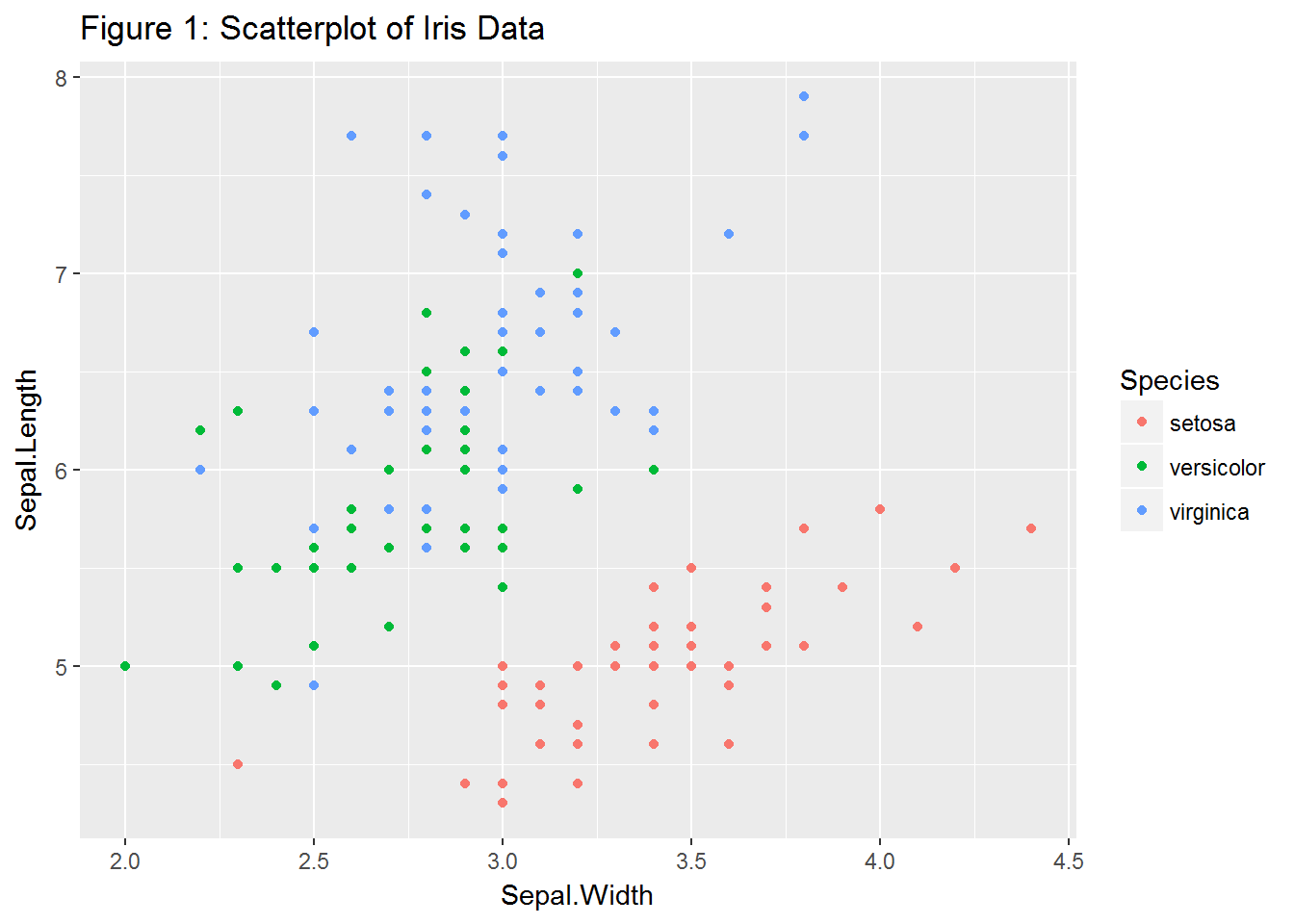Questions:

1. There are four potential explanatory variables in this data set. Modify Figure 1 by changing the two explanatory variables to create the most distinct species groups. In other words, create distinct clusters in which the species overlap as little as possible.
2. Create a new scatterplot with Petal.Width on the x-axis and Sepal.Width on the y-axis. Add two cut points (vertical lines) on the x-axis that break the predictor space (i.e. scatterplot) into three regions. Ideally, you should choose these regions such that each region contains only one species, though this is not always possible. After choosing these cut points, draw vertical lines visualizing your choices. If you are using the ggformula package, you can use gf_vline(xintercept = ___) to draw these lines.
3. Based upon the vertical lines created in Question 2, write mathematical rules to identify the three regions. For example, when x is between 0 and 4, we are in the setosa region, when x is between 4 and 5 we are in the versicolor region. These are called spliting rules and will be used to identify regions corresponding to specific species within our decision tree.

In the previous questions you:

• Used visual inspection to determine which of the four explanatory variables was best in properly classifying the response variable. Later we will describe algorithms to identify which variables to use.
• Developed splitting rules based on this one explanatory variable. Typically CART analysis involves multiple explanatory variables.
• Created a one dimensional (i.e. one explanatory variable) predictor space. This predictor space is used to make predictions about the response variable. An n-dimensional predictor space is a region created by splitting rules on n explanatory variables.

2. Creating Classification Trees

Splitting on One Variable

To create a tree diagram using only the Petal.Width variable, we utilize the tree() function in the tree package. As done in Questions 1 - 3, we will create mathematical rules to divide the predictor space into distinct, non-overlapping regions. Then, every observation within a particular region is given the same predicted value.

The technique to create these classification trees is often called recursive binary splitting. This is called binary because we are splitting the predictor space into two pieces and called recursive because we repeat this process multiple times. In essence, classification trees are created by:

• finding a single node that maximizes the accuracy and then splitting the data based upon this node
• using the split data, finding the second best node to split the data
• repeating the process until we hit an appropriate stopping point.
#Create a tree diagram with Species as the predicted value and Petal.Width as the explanatory variable.
tree1 <- tree(Species ~ Petal.Width, data = iris)
plot(tree1)
text(tree1)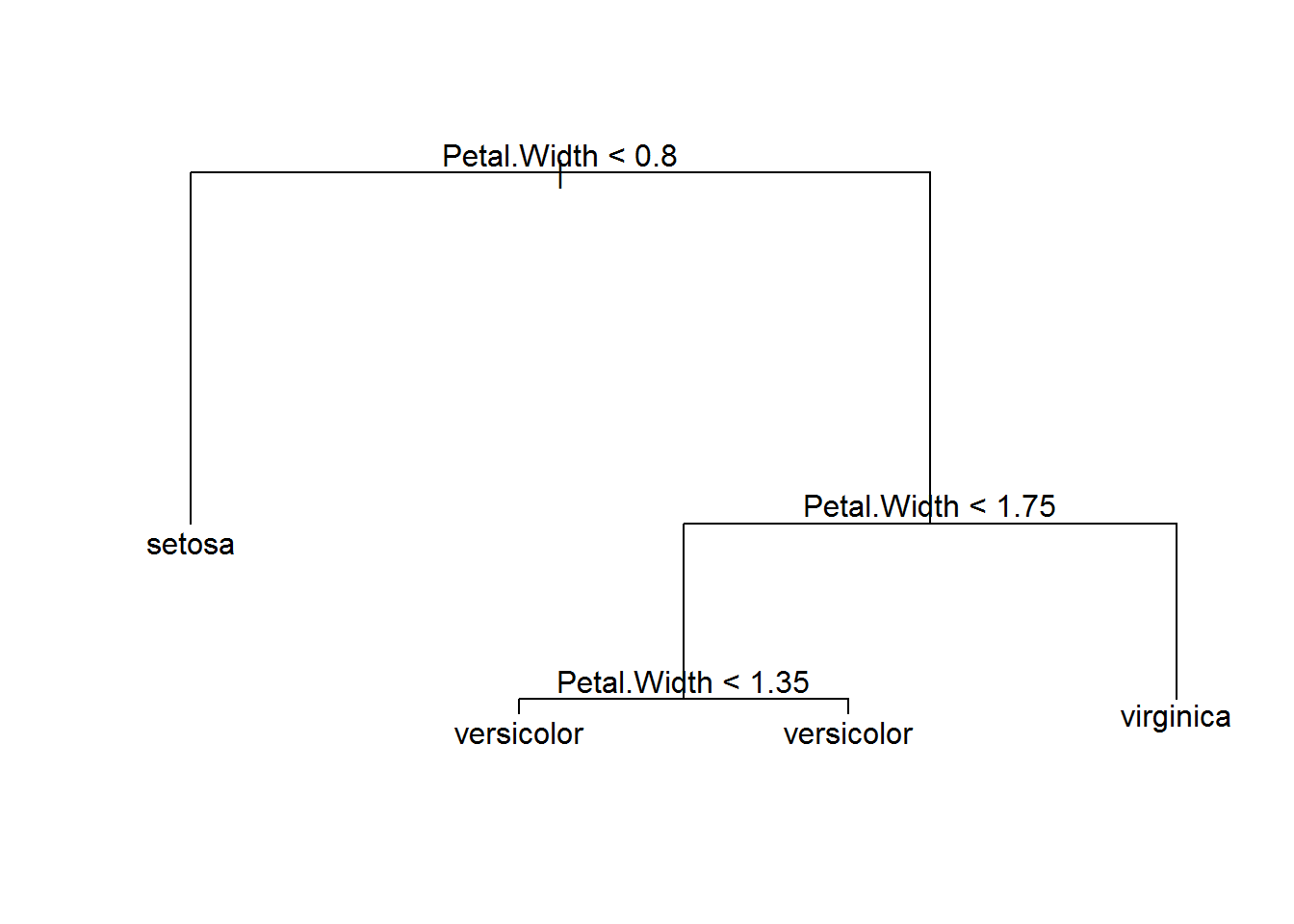The classification tree consists of nodes that some say make it look like an upside down tree. Internal nodes have exactly one incoming edge and then the data are split based upon a decision (a mathematical rule to split the predicted response). All other nodes are called leaves (also known as terminal nodes or decision nodes).

• The tree() function reads the iris data and determines the first splitting rule to be at Petal.Width = 0.8. We predict that when Petal.Width < 0.8, the species will be setosa.
• When Petal.Width > 0.8, we create a second slitting rule. We classify the species as versicolor when 0.8 < Petal.Width < 1.75 and any point with Petal.Width > 1.75 will be classified as virginica.
• Note that the final splitting rule based upon Petal.Width = 1.35 can be ignored, since it does not actually make any impact on our decision rules.

There are multiple ways to assess the accuracy of decision trees. One of the most straight forward approaches is to simply count the number of points that were misclassified. Here we use the summary() function to calculate that our misclassification rate = 6/150 = 4%.

summary(tree1)
##
## Classification tree:
## tree(formula = Species ~ Petal.Width, data = iris)
## Number of terminal nodes:  4
## Residual mean deviance:  0.2404 = 35.09 / 146
## Misclassification error rate: 0.04 = 6 / 150

Review the graph you created in Question 2 to verify that, for any vertical lines selected, at least 6 points will be misclassified. Notice that there are multiple rules that could be used that would give an identical misclassification rate. For example, creating our first split at Petal.Width = 0.6 or Petal.Width = 0.9 would have the same misclassification rate.

Splitting on Multiple Variables

To visualize how to use two explanatory variables to create a classification tree, go to the CART Shiny App. Try to determine the best splitting rules for the iris data. In the app, use the Iris1 data set. Select the splitting rule for the x-axis by using the x slider. To split the data on the y-axis, select the appropriate box and move the corresponding y slider. Note that the accuracy rate and optimized tree can also be shown.

The tree() function can easily be modified to incorporate more than one explanatory variable. Below we use ~ Petal.Length + Petal.Width to identify specific explanatory variables to include in our tree. We can also use ~ . to construct a classification tree which could potentially use all the explanatory variables. Note that the tree() function doesn’t necessarily use all the explanatory variables. Instead, this algorithm selects only variables that are useful in the classification process.

tree2 <- tree(Species ~ Petal.Length + Petal.Width, data = iris)
plot(tree2)
text(tree2)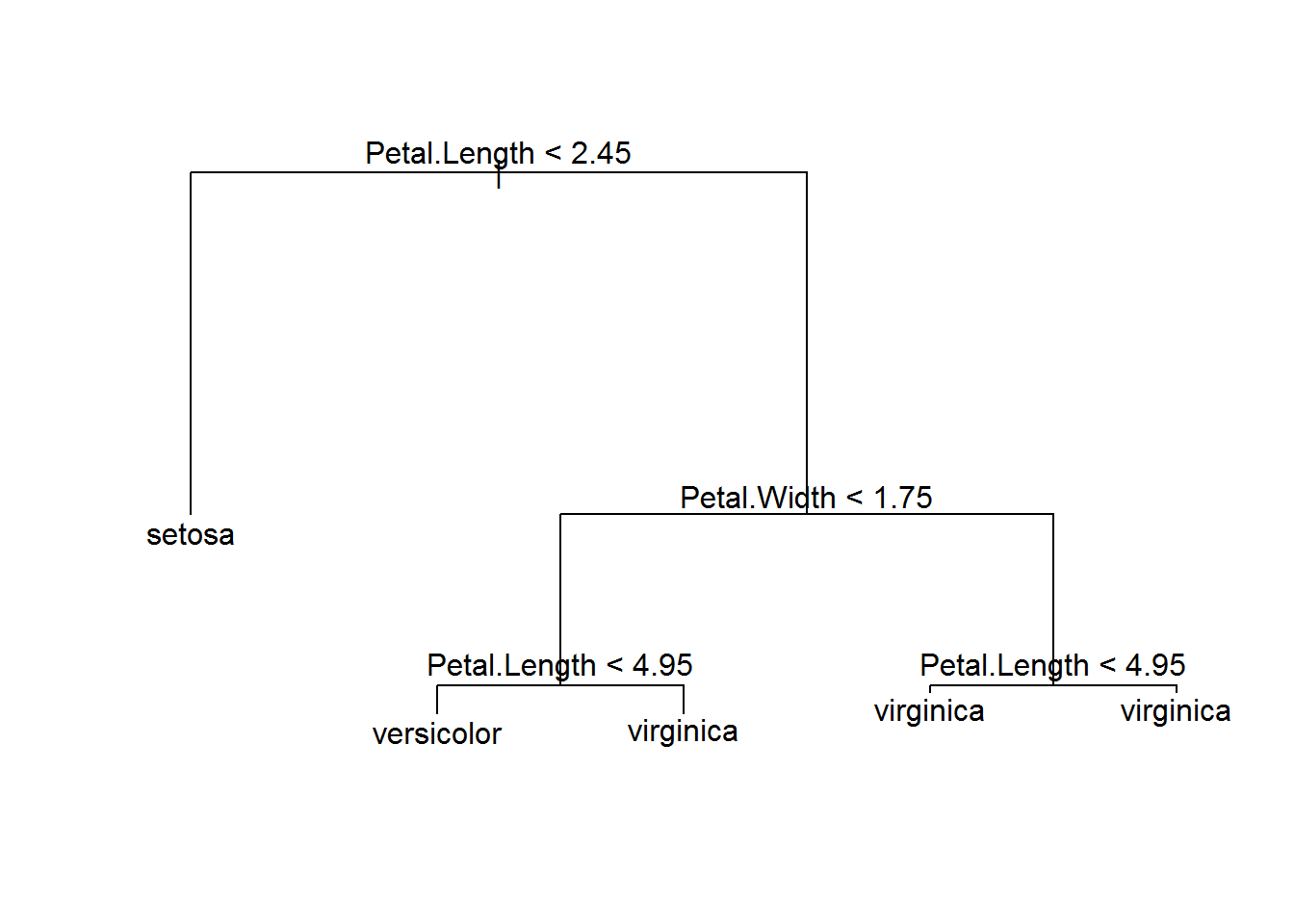treeall <- tree(Species ~ . ,data = iris)
plot(treeall)
text(treeall)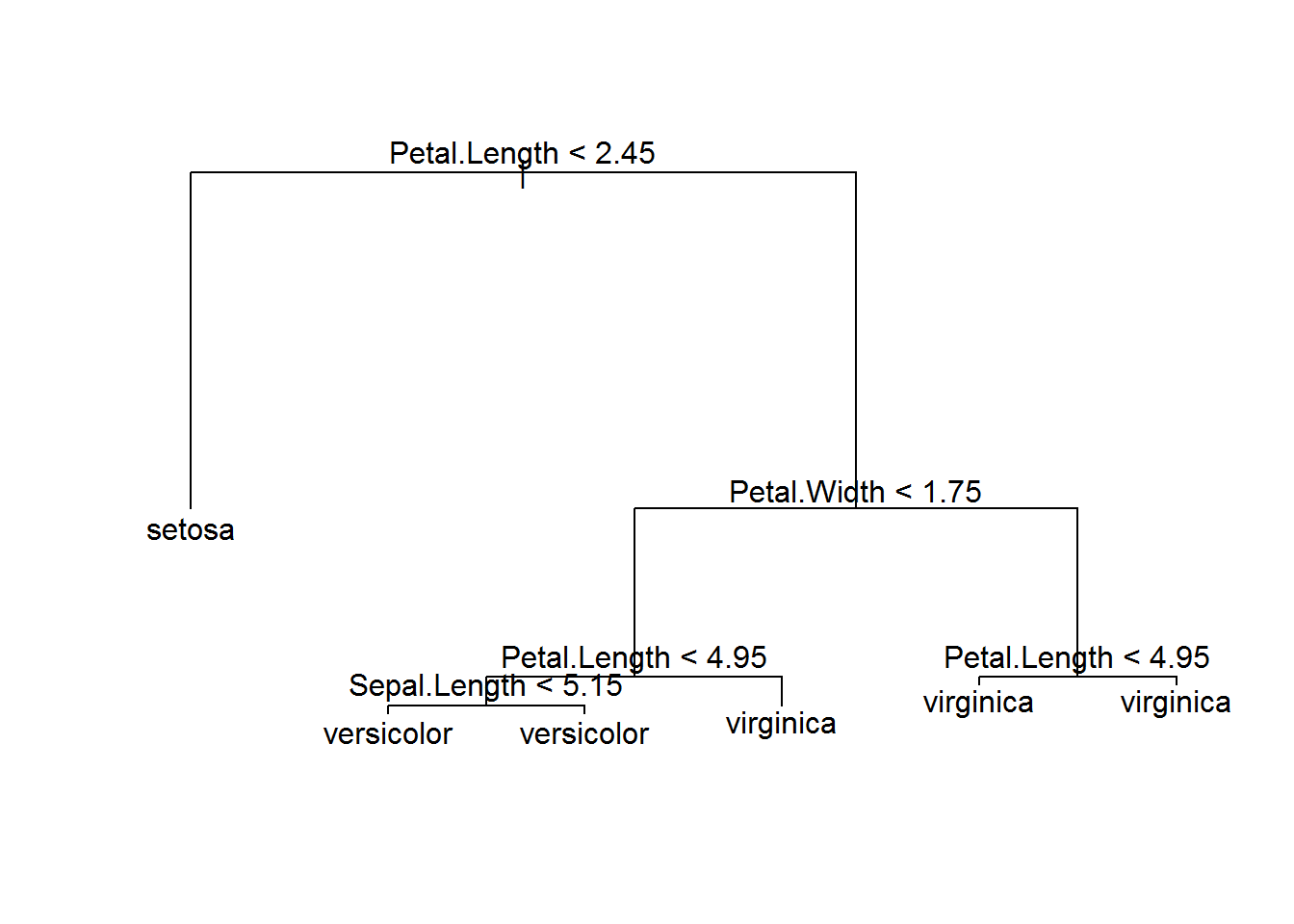Questions:

1. Using the tree2 model, if Petal.Width = 1.5 and Petal.Length = 5, what would you classify the species to be?
2. Use the summary() function on both the tree2 and treeall models and give their misclassification rate. Compare both classification trees and why you may prefer to use the tree2 model instead of the treeall model.

3. How are CART Models Evaluated?

The trees shown above can quickly get very complex. We are often interested in limiting the number of nodes while still getting accurate predictions. One way to measure accuracy is to simply count misclassification rate. We then create a table of actual versus predicted values to determine our misclassification rate.

# Create a summary table (called a confusion matrix)
#output is the class instead of the probability
tree.pred <- predict(tree1, iris, type = "class")
table(tree.pred, iris\$Species)
##
## tree.pred    setosa versicolor virginica
##   setosa         50          0         0
##   versicolor      0         49         5
##   virginica       0          1        45

Questions:

1. Using the table of actual versus predicted values for the tree1 model, how many misclassifications were made? How many virginica species were misclassified as versicolor?
2. Create your own confusion matrix using the treeall model. How many versicolor were misclassified as virginica?

The misclassification rate is a straight-forward measure of the accuracy of our model predictions. However, with large data sets, other measures such as the Gini impurity or cross entropy, are typically used when developing classification trees (James et. al., 2013).

4. Cross Validation

The previous sections describe how to create classification trees. However, the trees we created did not provide very much useful information, since we already know the actual species for all 150 plants in our data set. Typically the goal of CART is to make predictions, i.e. when we have the explanatory variables for new plants and we want to be able to accurately predict the unknown response.

To test a model’s ability to make accurate predictions with new data, researchers typically fit a model using only a sample of their original data. This reduced data set is often called a training data set. After a model is fit using their training data set, researchers test their model on the remaining data, often called the testing data set or holdout data set. This technique is called cross validation. Cross validation is essential for properly evaluating the performance of a model. There are many types of cross validation techniques based upon how the training data is selected. We demonstrate one example of the cross validation process below.

# we use set.seed to ensure that the same random sample is selected every time. This is only for demonstration purposes to ensure everyone will get exactly the same answer with this code.
set.seed(123)
# Randomly sample 100 rows from the iris data set. These 100 rows will be used as our training data set.
train <- sample(1:nrow(iris), 100)
# Create a classification tree using only the training data
tree.iris <- tree(Species ~ ., iris, subset = train)
plot(tree.iris)
text(tree.iris)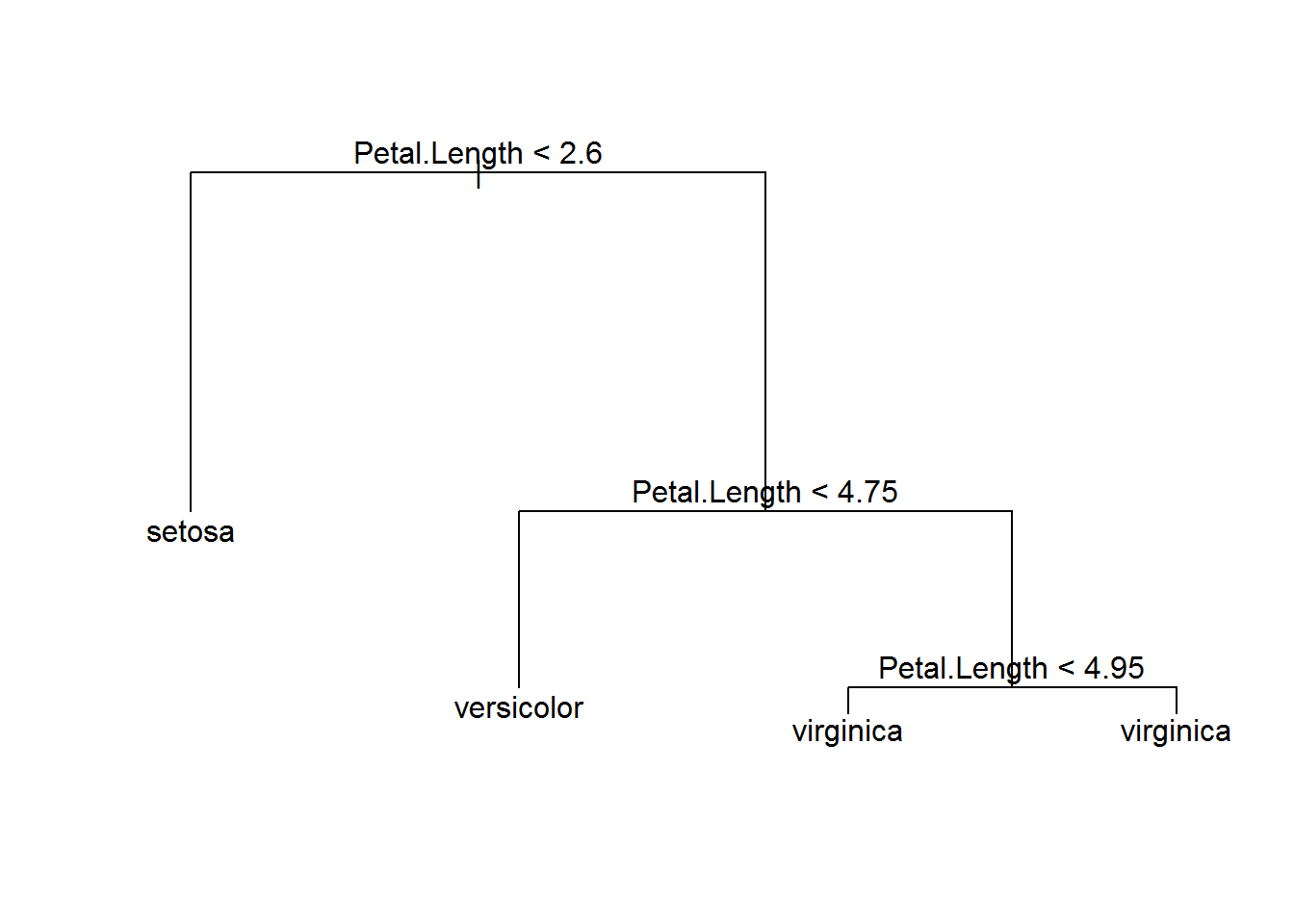# Use the tree.iris model on the testing data, iris[-train],
tree.pred <- predict(tree.iris, newdata = iris[-train,], type = "class")
# Create a summary table of our results based on the testing data
with (iris[-train,], table(tree.pred, Species))
##             Species
## tree.pred    setosa versicolor virginica
##   setosa         14          0         0
##   versicolor      0         16         1
##   virginica       0          3        16

Questions

1. In the example above, what is the misclassification rate for the training data? What is the misclassification rate for the testing data?
2. In general, would you expect the misclassification rate to be higher in the training or the testing data? Briefly explain why.
3. Why does changing the seed create different misclassification rates?

In the above example, we used two thirds of our data (100 rows) for training and one third of our data (50 rows) for testing. There is no exact rule for the percentage to use for the training data set. Many researchers suggest using 80% of your data for training, while others suggest using between 50% to 90% of the data for training. This decision will depend on the size and complexity of your data. In general, more training data will allow the researcher to create better models and more testing data is useful to accurately evaluate those models. It is common to have thousands of rows of data when creating classification trees. Data sets with only a few hundred rows are rarely useful, especially if there are a large number of explanatory variables.

5. Stopping Rules

Whenever researchers create models, they must consider trade-offs between cost (loss in model accuracy) and complexity. For any data set, it is possible to construct a series of mathematical rules that will create a model that is 100% accurate when the response variable is provided within the data. However, that model typically

• makes very poor predictions with new data (or testing data) and
• is too complex for practical use.

Models are over fit when they are too focused on the current data set. That is, in order to increase the accuracy of their model, complex models may sometimes be built to account for random noise in the data. Consequently, an over-fit model produces accurate classifications for the training set, but makes inaccurate predictions for a new data, such as the test set.

To create a parsimonious model (a model that carefully selects a relatively small number of the most useful explanatory variables), researchers create rules to stop making additional nodes, known as stopping rules. For example, if a model is too complex (the tree has too many nodes), researchers often want to “prune” the tree. Below we use two functions, cv.tree() and prune.tree(), to demonstrate a cross-validation method to create stopping rules.

cv.iris <- cv.tree(tree.iris, FUN = prune.misclass)
plot(cv.iris)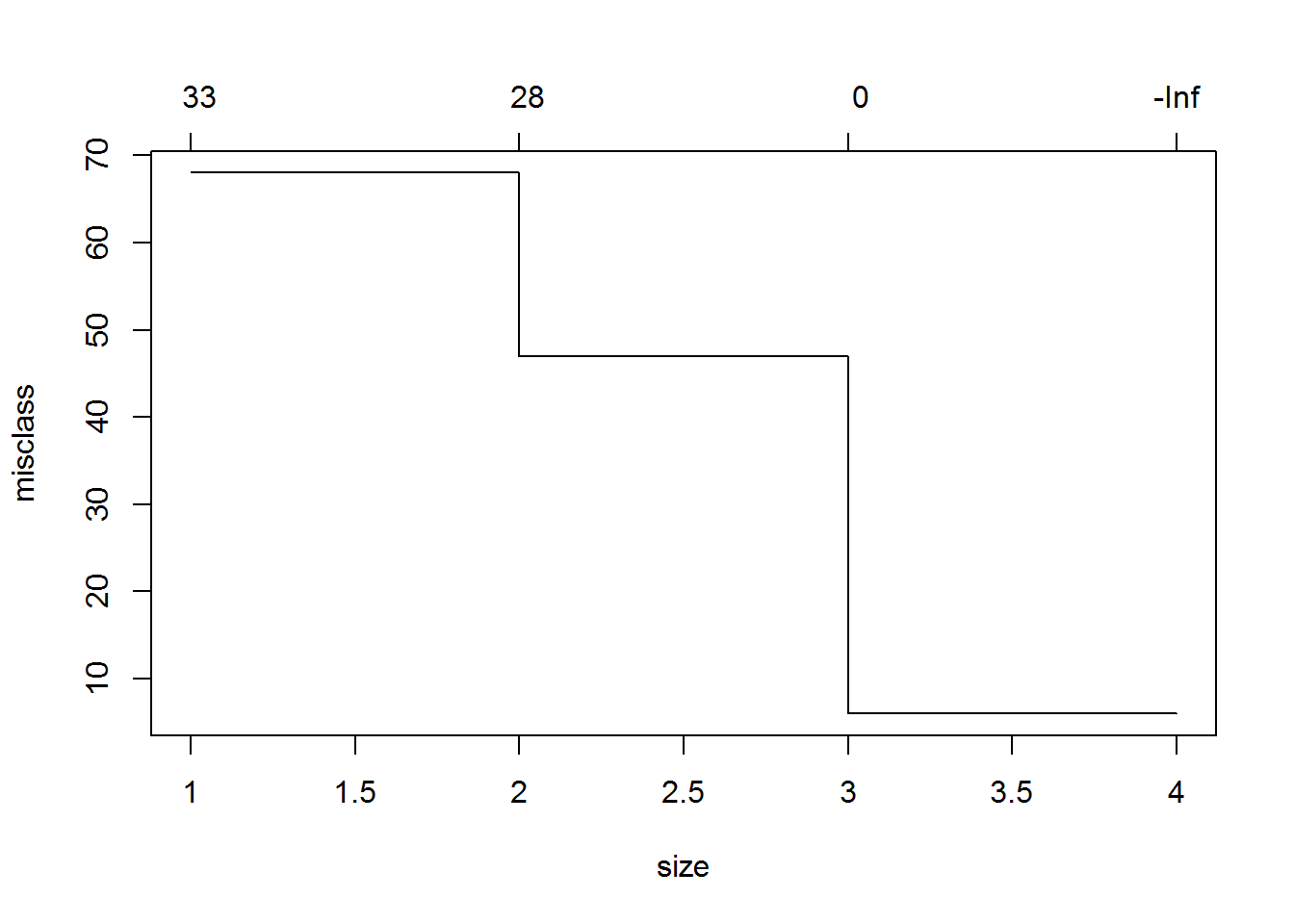In general, we want to select a tree size that corresponds to the smallest amount of overall errors when using cross validation. The plot of cv.iris tells us that the size (the number of terminal nodes) on the x axis and the misclassification rate on the y axis. In our example, we see that 3 or 4 terminal nodes corresponded to the lowest error rates. In other words, a model with 3 terminal nodes is the simplest model that still has low misclassification rates. Note that while we used the misclassification rate, FUN = prune.misclass to make decisions on pruning this tree, other measures of accuracy are often used. In the code below we create a tree where the number of terminal nodes is set to 3.

prune.iris <- prune.misclass(tree.iris, best = 3)
plot(prune.iris)
text(prune.iris)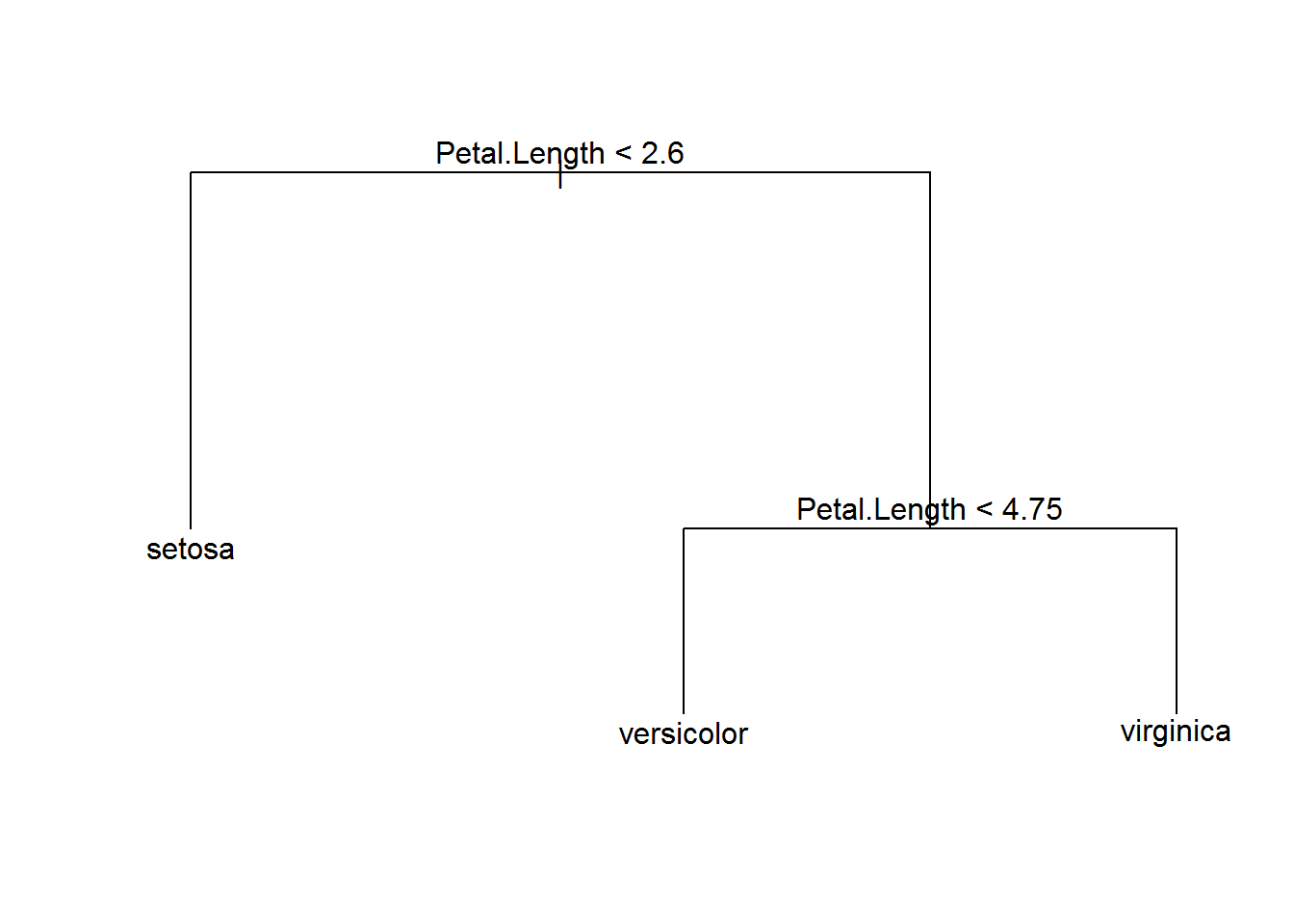Important Note The above code uses cross validation methods on the training data to determine the appropriate size of the tree. In other words, the training data is again subsetted into new training and testing data sets, trees are created, and error rates are calculated. This process occurs multiple times, each with new randomly selected training and testing data sets, to give a measure of the expected error rate for each tree size. In our example, this pruning process is based on our original 100 rows of training data. Testing data should never be used in the creation of a model.

After we have developed a final model based on the training data, we then use the testing data one time to get a measure of the accuracy of our model. If the testing data is used anywhere in the process of model development, the confusion matrix is not a true measure of our ability to accurately make predictions on new data.

Below we create a confusion matrix to evaluate the accuracy of our final pruned model, prune.iris.

tree.pred <- predict(prune.iris, iris[-train,], type = "class")
with (iris[-train,], table(tree.pred, Species))
##             Species
## tree.pred    setosa versicolor virginica
##   setosa         14          0         0
##   versicolor      0         16         1
##   virginica       0          3        16

Important considerations when using CART

• Trees tend to over fit data, so small changes to input data could result in major changes to tree structure.
• CART can use the same variables more than once in different parts of the tree.
• On its own, CART is not the best type of supervised learning technique. More advanced techniques, such as Random Forests and Boosting are often suggested.
• There are other, more recent, implementations of CART in R. For example, rpart package provides a much faster implementation than the tree package, and the package party creates much better visualizations. If you are conducting an actual research project using CART analysis, please consider one of these packages.
• In this example, the explanatory variables are quantitative, but categorical explanatory variables can also easily be incorporated into a tree.
• The process for creating regression trees (quantitative responses) is very similar to classification trees. Both methods use recursive binary splitting to create nodes to form a tree. This process is repeated until we reach an appropriate stopping point. The key difference is based on how we measure the accuracy of the tree. With categorical responses, we create nodes that minimize the misclassification rate. With quantitave response data, we create nodes that minimize the squared difference between the predicted and actual values.